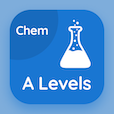Cambridge Online Courses (GCE)

A Level Chemistry Quizzes

A Level Chemistry Quiz PDF - Complete

# Order of Reaction Multiple Choice Questions p. 116

Study Order of Reaction multiple choice questions and answers, order of reaction quiz answers PDF 116 to study A Level Chemistry course online. Reaction Kinetics MCQ trivia questions, order of reaction Multiple Choice Questions (MCQ) for online college degrees. "Order of Reaction MCQ" PDF eBook: equilibrium and solubility, sub shells and atomic orbitals, electrolysis process, balancing equations: reactions with oxygen, order of reaction test prep for online bachelor degree programs.

"For the first and second order of reactions, the graph will be" MCQ PDF: falling, straight line, rising, and curve for ACT test prep classes. Learn reaction kinetics questions and answers to improve problem solving skills for college entrance exams.

## Order of Reaction Questions and Answers MCQs

MCQ: For the first and second order of reactions, the graph will be

straight line
falling
rising
curve

MCQ: Consider the reaction: Na(s) + O2(g) → Na2O(s). Moles of sodium needed to balance the equation would be

1
2
3
4

MCQ: In electrolysis of pure ionic molten compounds, metal is formed at

anode
cathode
inert electrode
base of the apparatus

MCQ: The shape of s-orbital is

tetrahedral
octagonal
spherical
round

MCQ: When an undissolved ionic compound comes in contact with a saturated solution, there is an establishment of

stability
constancy
equilibrium
anion

### More Quizzes from A Level Chemistry Course